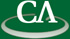The Exact Solution of Trefethen's 100 digit Challenge Problem Number 7

Greg Fee, CECM

Thursday May 25th, 2006 at 10:30am in K9509.

```Abstract:

In the January 2002 edition of SIAM News,
Nick Trefethen announced the "\$100, 100-Digit Challenge".
Each problem had a solution that was a single real number.
We were only asked to obtain the first 10 significant digits
of the solution to each problem, for a total of 100 decimal digits.
Problem number 7 was to find the [1,1] element of the inverse
of an integer matrix. The exact solution is a rational number.
This exact rational number was first obtained using LinBox.
We show how to obtain the exact solution using Maple10 .

```